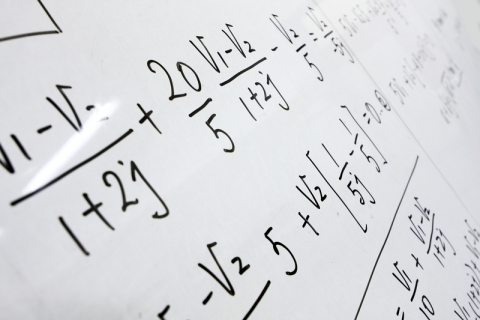### Use your skills in problem solving to solve some of society's biggest problems

Welcome to the School of Maths and Physics. Do you have a head for numbers and a talent for solving problems? Math courses give students the mathematical, analytical and problem-solving skills needed to solve some of society's biggest problems, from predicting the spread of deadly diseases, to how to make the perfect cup of coffee.

## Mathematician Heros - Solving the World’s problems

Maths isn’t just theoretical. Mathematical principles govern everything in our lives. This means that mathematicians can help solve a really varied range of problems, many of which relate to everyday life.

Click on the boxes below to learn about some of the interesting and unusual topics mathematicians at the University of Portsmouth work on.## Predicting the spread of disease

One important use of mathematics is in the prediction of how deadly diseases might spread - using the principle of modelling. You’ll have seen some examples of this during the Government briefings on covid-19, but here is your chance to have a go at Modelling yourself.

You can click through the slides in this interactive activity to: